# How to predict with missing values ?¶

In this tutorial, we will cover how to accurately predict the response y given X when X contains missing values.

In :
import numpy as np

import matplotlib.pyplot as plt
%matplotlib inline


# 1 Get your data (with missing values)¶

In :
# Load you data here

from sklearn.datasets import fetch_california_housing
X_complete, y = fetch_california_housing(return_X_y = True)

In :
# Artificially add some np.nan

np.random.seed(1)  # fix the seed

def ampute_mcar(X_complete, y=None):
# Add completly at random missing values

X = X_complete.copy()
return X

def ampute_mnar(X_complete, y=None):
# Add missing values not at random

X = X_complete.copy()
missing_mask = (np.random.normal(X_complete) > X_complete + 1)

if y is not None:
## Add missing values correlated with y
y_max = np.percentile(y, 90)

return X

In :
X_mcar = ampute_mcar(X_complete)
X_mnar = ampute_mnar(X_complete, y)
X = X_mcar


### Quick Exploratory Data Analysis¶

Few lines of code to appreciate the missingness in our data

In :
print('Shape of X:', X.shape)

Shape of X: (20640, 8)

In :
np.set_printoptions(precision=4, suppress=True) #  supress scientific notation
(X[:5])

Out:
array([[   8.3252,   41.    ,    6.9841,    1.0238,  322.    ,    2.5556,
37.88  , -122.23  ],
[   8.3014,   21.    ,    6.2381,    0.9719, 2401.    ,       nan,
37.86  , -122.22  ],
[   7.2574,   52.    ,    8.2881,    1.0734,       nan,       nan,
37.85  , -122.24  ],
[      nan,       nan,    5.8174,    1.0731,  558.    ,       nan,
37.85  , -122.25  ],
[      nan,   52.    ,    6.2819,    1.0811,  565.    ,       nan,
37.85  , -122.25  ]])
In :
print('Ratio nan in X:', np.round(100 * np.sum(np.isnan(X)) / X.size,1), '%')
print('Ratio nan in y:', np.round(100 * np.sum(np.isnan(y)) / y.size,1), '%')

Ratio nan in X: 20.0 %
Ratio nan in y: 0.0 %

In :
plt.scatter(X[:400,0], X[:400,1]);  #  data with missing coordinate are not plotted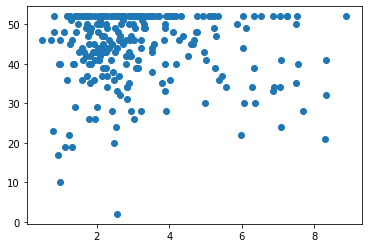# 2 Imputation Model¶

Our goal is to predict y as accurately as possible. There exists mainly two options to deal with missing values:

1. Either we get rid of all missing values (ease of use at the price of loosing information);
2. Or we impute the missing values.

We will describe the second option in details.

## Imputation strategies¶

Since there is no prefer way to deal with missing values, we will consider three imputation strategies:

1. Mean imputation: replace nan by the mean [or the median] of the feature (the column);
2. Iterative imputation: each feature is regressed/estimated from the others;
3. MinMax Imputation (also refer as Missing In Attribute, by Twala et al.): duplicating features twice and replace its missing values once by $\infty$ and once by $- \infty$.

In the case of Mean or Iterative imputation, it might by fruitfull to add the missing values indicator (by concatenating the missing indicator to $X$)

Rule of thumb: If we possess a good learner algorithm and enough samples in our dataset (e.g. more than $10^5$), the mean imputation is a good default choice (since it is consistent).

In :
from sklearn.impute import SimpleImputer

imputer = SimpleImputer(strategy='mean')
X_impute = imputer.fit_transform(X)
print(X_impute[:5])

[[   8.3252   41.        6.9841    1.0238  322.        2.5556   37.88
-122.23  ]
[   8.3014   21.        6.2381    0.9719 2401.        3.1038   37.86
-122.22  ]
[   7.2574   52.        8.2881    1.0734 1428.183     3.1038   37.85
-122.24  ]
[   3.8718   28.674     5.8174    1.0731  558.        3.1038   37.85
-122.25  ]
[   3.8718   52.        6.2819    1.0811  565.        3.1038   37.85
-122.25  ]]

In :
print('Ratio nan =', np.round(100 * np.sum(np.isnan(X_impute)) / X.size,1), '%')

Ratio nan = 0.0 %

In :
plt.scatter(X_impute[:400,0], X_impute[:400,1])

missing_mask = np.where([any(x) for x in np.isnan(X[:400][:,[0,1]])])

Out:
<matplotlib.collections.PathCollection at 0x7f087a881940>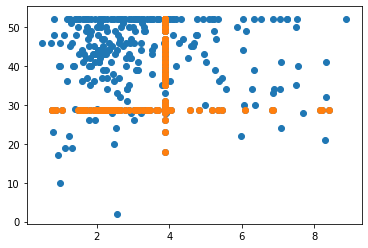In :
# explicitly require because IterativeImputer is still experimental in sklearn
from sklearn.experimental import enable_iterative_imputer
from sklearn.impute import IterativeImputer

imputer = IterativeImputer()
X_impute = imputer.fit_transform(X)
print(X_impute[:5])

[[   8.3252   41.        6.9841    1.0238  322.        2.5556   37.88
-122.23  ]
[   8.3014   21.        6.2381    0.9719 2401.        9.2866   37.86
-122.22  ]
[   7.2574   52.        8.2881    1.0734  408.2665    0.8595   37.85
-122.24  ]
[   4.5338   33.2761    5.8174    1.0731  558.       -3.1331   37.85
-122.25  ]
[   4.824    52.        6.2819    1.0811  565.        1.3492   37.85
-122.25  ]]

/home/nicolas/anaconda3/lib/python3.6/site-packages/sklearn/impute/_iterative.py:603: ConvergenceWarning: [IterativeImputer] Early stopping criterion not reached.
" reached.", ConvergenceWarning)

In :
plt.scatter(X_impute[:400,0], X_impute[:400,1])

missing_mask = np.where([any(x) for x in np.isnan(X[:400][:,[0,1]])])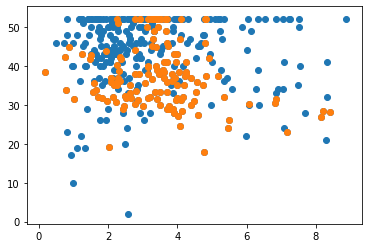Imputation here takes advantage of others features.

# 3. Simulations/Experiments¶

Let's evaluate the differents imputation strategies. Let's consider our differents imputers w.r.t. differents machine learning algorithms. The pipeline will be

1. Imputation
1. Regression on the imputed dataset

### 3.1 Step by step pipeline with LinearRegression()¶

Here we decompose each step of the pipeline for clarity.

In :
# First split the data into train set and test set
from sklearn.model_selection import train_test_split
X_train, X_test, y_train, y_test = train_test_split(X, y)
print('train shape: X.shape={}, y.shape={}'.format(X_train.shape, y_train.shape))
print('test shape: X.shape={}, y.shape={}'.format(X_test.shape, y_test.shape))

train shape: X.shape=(15480, 8), y.shape=(15480,)
test shape: X.shape=(5160, 8), y.shape=(5160,)

In :
from sklearn.linear_model import LinearRegression
regressor = LinearRegression()

In :
""" Line below would raise an ValueError:
'Input contains NaN, infinity or a value too large for dtype('float64').'
Because LinearRegressor couldn't deals with NaN values.""";

# regressor.fit(X_train, y_train)

In :
# Good practice are: fitting on the train, then transform both train and test with the *same* imputer

imputer = SimpleImputer(strategy='mean')
imputer.fit(X_train)

X_train_imp = imputer.transform(X_train)
X_test_imp = imputer.transform(X_test)

In :
regressor.fit(X_train_imp, y_train)
print('Score on train:', regressor.score(X_train_imp, y_train))
print('Score on test:', regressor.score(X_test_imp, y_test))

Score on train: 0.43620914183706777
Score on test: 0.4264164447205786

In :
# Try with the IterativeImputer now
imputer_it = IterativeImputer()
imputer_it.fit(X_train)

X_train_imp_it = imputer_it.transform(X_train)
X_test_imp_it = imputer_it.transform(X_test)

regressor.fit(X_train_imp_it, y_train)
print('Score on train:', regressor.score(X_train_imp_it, y_train))
print('Score on test:', regressor.score(X_test_imp_it, y_test))

Score on train: 0.5016123112292037
Score on test: 0.48840890705990336

/home/nicolas/anaconda3/lib/python3.6/site-packages/sklearn/impute/_iterative.py:603: ConvergenceWarning: [IterativeImputer] Early stopping criterion not reached.
" reached.", ConvergenceWarning)


Here we see that the Iterative imputation method can add information for Linear Regression method.

### 3.2 Model selection¶

Now we run our pipeline on severals imputers and regressors.
We will use Pipeline from sklearn to sequentially apply imputation and regression.
We finally use sklearn cross_val_score to evaluate our models.

In :
# Imputer model:

from sklearn.preprocessing import MinMaxScaler
from sklearn.base import BaseEstimator, TransformerMixin

class MinMaxImputer(BaseEstimator, TransformerMixin):
# replacing the nan by two extremes values (by duplicating each column)

def fit(self, X, y=None):
mm = MinMaxScaler().fit(X)
self.data_min_ = mm.data_min_
self.data_max_ = mm.data_max_
return self

def transform(self, X):
X_min, X_max = X.copy(), X.copy()

for feature_idx in range(X.shape):
X_min[nan_mask, feature_idx] = self.data_min_[feature_idx] - 1
X_max[nan_mask, feature_idx] = self.data_max_[feature_idx] + 1

return np.concatenate([X_min, X_max], axis=1)

'MinMax': MinMaxImputer()}

In :
# Regressor model:

from sklearn.linear_model import LinearRegression
from sklearn.svm import SVR
from sklearn.ensemble import RandomForestRegressor
from sklearn.ensemble import HistGradientBoostingRegressor  # similar to XGBoost/lightGBM

regressors = {'LinReg': LinearRegression(),
#               'SVM': SVR(C=100),
'RandomForest': RandomForestRegressor(),

In :
from sklearn.pipeline import Pipeline
from sklearn.model_selection import ShuffleSplit, cross_val_score

def plot_score_regressor(X, y, regressor, regressor_name='regressor', X_complete=None):
# Boxplot scores for each imputation strategies

l_score = []
for imputers in [imputers_wo_indicator, imputers_with_indicator]:
for imputer_name, imputer in imputers.items():
pipeline = Pipeline([(imputer_name, imputer), (regressor_name, regressor)])
score = cross_val_score(pipeline, X, y, n_jobs=-1, cv = 5)
l_score.append(score)

score_ground_truth = cross_val_score(regressor, X_complete, y, n_jobs=-1, cv = 5)
l_score.append(score_ground_truth)
plt.title(regressor_name)
bplot = plt.boxplot(l_score[::-1], labels=labels[::-1],
vert=False, patch_artist=True)

for patch, color in zip(bplot['boxes'],
['C0','C1','C2','C3', 'C4', 'C5']):
patch.set_facecolor(color)

In :
%%time
for regressor_name, regressor in regressors.items():
plt.figure(figsize=(15,5))
for ii, (X, X_name) in enumerate(zip([X_mcar, X_mnar], ['MCAR', 'MNAR'])):
plt.subplot(1, 3, ii+1)
plot_score_regressor(X, y, regressor, regressor_name + ' ' + X_name, X_complete)

/home/nicolas/anaconda3/lib/python3.6/site-packages/joblib/externals/loky/process_executor.py:706: UserWarning: A worker stopped while some jobs were given to the executor. This can be caused by a too short worker timeout or by a memory leak.
"timeout or by a memory leak.", UserWarning

CPU times: user 1.21 s, sys: 165 ms, total: 1.38 s
Wall time: 6min 46s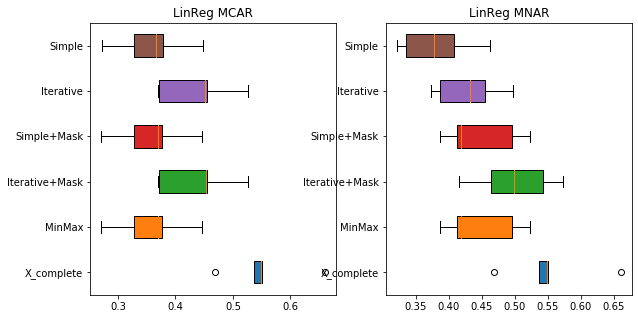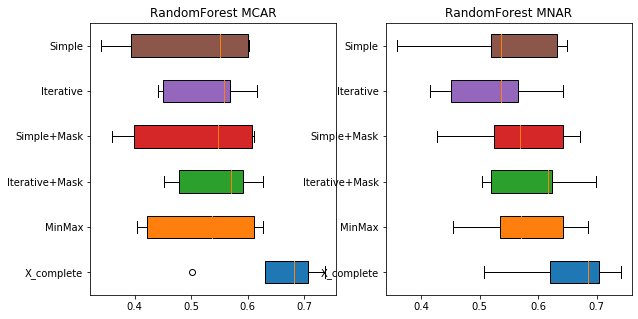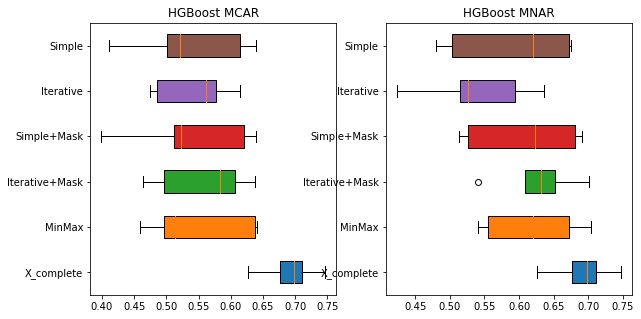Note that in the HistGradientBoostedTree, MIA (i.e. replacing the np.nan by the two extremes values, by duplicating each column containing np.nan) will be implemented by default in the next release of sklearn in HistGradientBoostedTree

# Summary¶

• To train and test on data with missing values, the same imputation model should be used.

• Missing Incorporated in Attribute (MIA) is a good solution for tree-based models (e.g. lightGBM implementation, sklearn next release). It handles missing values information.

• Imputation methods reduce the number of samples required to reach good prediction

• When missingness is related to the prediction target, it is useful to add indicator of missing entries as features